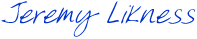The parallel tasks library was introduced with the .NET Framework 4.0 and is designed to simplify parallelism and concurrency. The API is very straightforward and usually involves passing in an `Action` to execute. Things get a little more interesting when you are dealing with asynchronous models such as events.

While the TPL has explicit wrappers for the asynchronous programming model (APM) that you can read about here: TPL APM Wrappers, there is no explicit way to manage events.

I usually hide the “muck” of subscribing and waiting for a completed action in events with a callback. For example, the following method generates a random number. I’m using a delay to simulate a service call and a thread task to make the call back asynchronous: you call into the method, then provide a delegate that is called once the information is available.

```private static void _GenerateRandomNumber(Action<int> callback)
{
var random = _random.Next(0, 2000) + 10;
Console.WriteLine("Generated {0}", random);
{
callback(random);
}
```

Now consider an algorithm that requires three separate calls to complete to provide the input values in order to compute a result. The calls are independent so they can be done in parallel. The TPL supports “parent” tasks that wait for their children to complete, and a first pass might look like this:

```private static void _Incorrect()
{

var start = DateTime.Now;

int x = 0, y = 0, z = 0;

() =>
{
Task.Factory.StartNew(() => _GenerateRandomNumber(result => x = result),
Task.Factory.StartNew(() => _GenerateRandomNumber(result => y = result),
Task.Factory.StartNew(() => _GenerateRandomNumber(result => z = result),
}).ContinueWith(t =>
{
var finish = DateTime.Now;
x, y, z,
x+y+z,
finish - start);
_Parallel();
});
}
```

The code aggregates several tasks to the parent, the parent then waits for the children to finish and continues by computing the time span and showing the result. While the code executes extremely fast, the result is not what you want. Take a look:

```Press ENTER to begin (and again to end)

Generated 593
Generated 1931
Generated 362
```

You can see that three numbers were generated, but nothing was computed in the sum. The reason is that for the purposes of the TPL, the task ends when the code called ends. The TPL has no way to know that the callback was handed off to an asynchronous process (or event) and therefore considers the task complete once the generate call finishes executing. This returns and falls through and the computation is made before the callback fires and updates the values.

So how do you manage this and allow the tasks to execute in parallel but still make sure the values are retrieved?

For this purpose, the TPL provides a special class called `TaskCompletionSource<T>`. The task completion source is a point of synchronization that you can use to complete an asynchronous or event-based task and relay the result. The underlying task won’t complete until an exception is thrown or the result is set.

To see how this is used, let’s take the existing method and fix it using the completion sources:

```private static void _Parallel()
{
{
};

var start = DateTime.Now;

var finish = DateTime.Now;
Console.WriteLine("Parallel: {0}+{1}+{2}={3} [{4}]",
finish - start);
}
```

First, I create an array of the task completions. This makes for an easy reference to coordinate the results. Next, I create an array of the underlying tasks. This provides a collection to pass to `Task.WaitAll()` to synchronize all return values before computing the result. Instead of using variables, the tasks now use the `TaskCompletionSource` to set the results after the simulated callback. The tasks won’t complete until the result is set, so all values are returned before the final computation is made. Here are the results:

```Generated 279
Generated 618
Generated 1013
Parallel: 618+279+1013=1910 [00:00:01.9981143]
```

You can see that all generated numbers are accounted for and properly added. You can also see that the tasks ran in parallel because it completed in under 2 seconds when each call had a 1 second delay.

The entire console application can simply be cut and pasted from the following code — there are other ways to chain the tasks and make the completions fall under a parent but this should help you get your arms wrapped around dealing with tasks that don’t complete when the methods return, but require a synchronized completion context.

```class Program
{
private static readonly Random _random = new Random();

static void Main(string[] args)
{
Console.WriteLine("Press ENTER to begin (and again to end)");

_Incorrect();

}

private static void _Incorrect()
{

var start = DateTime.Now;

int x = 0, y = 0, z = 0;

() =>
{
Task.Factory.StartNew(() => _GenerateRandomNumber(result => x = result),
Task.Factory.StartNew(() => _GenerateRandomNumber(result => y = result),
Task.Factory.StartNew(() => _GenerateRandomNumber(result => z = result),
}).ContinueWith(t =>
{
var finish = DateTime.Now;
x, y, z,
x+y+z,
finish - start);
_Parallel();
});
}

private static void _Parallel()
{
{
};

var start = DateTime.Now;

var finish = DateTime.Now;
Console.WriteLine("Parallel: {0}+{1}+{2}={3} [{4}]",
finish - start);
}

private static void _GenerateRandomNumber(Action<int> callback)
{
var random = _random.Next(0, 2000) + 10;
Console.WriteLine("Generated {0}", random);